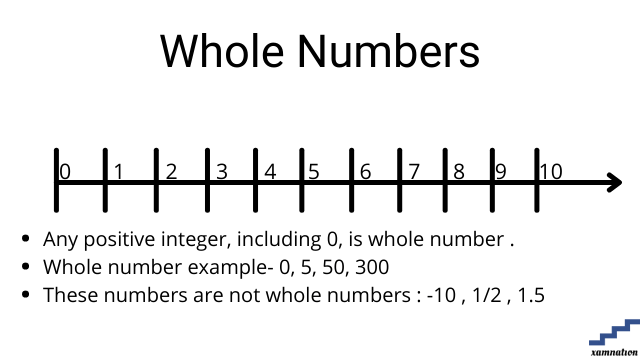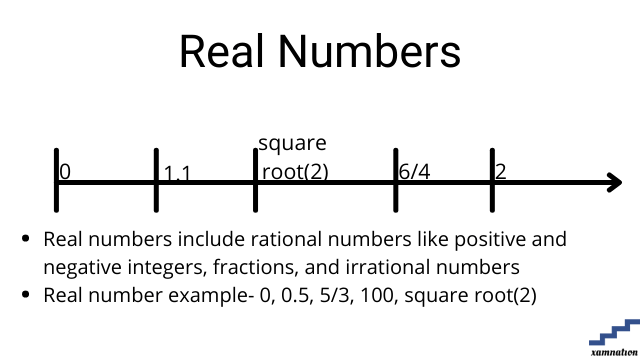# Maths for Grade 10 – Number System | what is real number | what is imaginary number | What is whole number

Number system tutorial

Number system is system of representing numbers in mathematics. Students deal with various types of numbers for solving mathematics formulas and calculation, data processing and handling complex topics like algebra and geometry.

In this article, we have shared different types of numbers that are used in mathematics. This article can be used by all grades students, as the definition of these different types of numbers is same for all grades.

## What is Whole Number?

### Definition of whole number

• Any positive integer (including 0) on the number line (up to infinity) is called whole number.
• It is positive number or 0
• It cannot be fraction, decimal or negative number### Examples of whole number

• 0 is a whole number
• 5, 10, 500, 3500 are whole numbers
• -5, 1.6 , 1/3 are not whole numbers

Practice question on whole number

Can you find whether below mentioned numbers are whole or not?

• 10
• 5
• 10/13
• -1.1
• -2/3
• 0
• 305

Send your solution of finding whole numbers to info@xamnation.com , and know detailed answer of this problem.

## What is Real Number?

### Definition of Real Number

• Real numbers include rational numbers like positive and negative integers, fractions, and irrational numbers
• Real number example- 0, 0.5, 5/3, 100, square root(2)
• Real number covers all whole number, rational, irrational , integer, decimals and fractions### Examples of Real Number

• 0, 1.1, 3/2 are example of real numbers
• All integer, decimal, rational ,irrational numbers are real numbers

Practice question on real number

Can you find whether below mentioned numbers is real number or not?

• 10
• 5
• -15
• 10/13
• -1.1

Send your solution of finding real number to info@xamnation.com , and know detailed answer of this problem.

## What is Imaginary number

### Definition of Imaginary number

• An imaginary number is a complex number that can be written as a real number multiplied by the imaginary unit i, which is defined by its property square(i) = −1
• Imaginary number example- 5i, 10i , -15i
• Imaginary numbers are not represented on real number line which mean they cannot be integer, whole number, rational, irrational , fraction or decimal### Examples of Imaginary number

• 5i, 2i are example of imaginary number.
• I is called iota which is square root of -1
• No real number is imaginary number

Practice question on Imaginary number

Can you find whether below mentioned numbers is Imaginary or not?

• 10
• 5
• 6i
• 10/13
• -1.1

Send your solution of finding imaginary number to info@xamnation.com , and know detailed answer of this problem.

## Conclusion

In this article, we have seen how to find whether number is real number, imaginary number and whole number. We also saw detailed examples and practical exercises for number system for grade 10 mathematics.

Are you struck in number system problem or any mathematics topics for grade 10 and above?

You can reach out to us for your math doubts and problem; our math tutors will help you in solving your doubts and take one-one math sessions online. Reach out to us, and our qualified math tutors for grade 6 and above will be glad to help you.